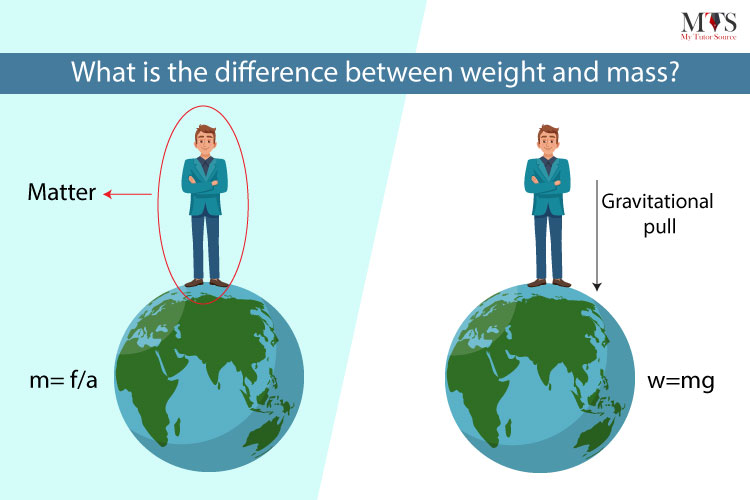# What Is the Difference Between Weight and Mass?

• Published On:
• Updated On:We often use Weight and Mass interchangeably which creates confusion in understanding the difference between these two values. Students often find mass and weight to be the same thing, however, it is really different and has their own importance in the history of Science.

## MASS:

Mass is the amount of matter present in an object or a substance. The standard unit (SI) of mass is Kilogram (Kg). However, smaller masses use units milligram (g). The mass stays the same everywhere, whether it is placed on the Earth or Moon or any other planet. For instance, if the mass of a specific object is 50Kg on Earth then even if it is placed on the Moon it will stay constant. Mass is denoted by ‘ m ’. There are many ways to calculate the mass of an object. Formulas to calculate the mass are the following:

Mass = Density (ρ) x Volume (V)

Mass = ρxV

Mass = Weight (W) ➗  Gravitational acceleration (g)

Mass = W/g

Mass = Force (F) ➗ Acceleration (a)

Mass = F/a

One of the important things about mass is that it can never be zero (0) and since it is constant everywhere it does not depend on gravity. There are many ways to measure mass of an object and some of them are through using ordinary balance. For instance, in scientific experiments, labs etc, balances like an electronic balance, double pan balance, lever balance, and triple beam balance etc. Mass is a base quantity. Moreover, Mass is a scalar quantity since it has only magnitude unlike Weight.

## WEIGHT:

Weight of an object is the measure of the gravitational pull on an object. The standard unit (SI) of weight is Newton (N). Unlike mass, weight does not stay the same anywhere, whether an object is placed on the Earth or Moon or any other planet. For instance, if the weight of a specific object or a person is 490 N (50kg) on Earth then if it is placed on Mars it will change because of the gravity being 3.7 there, and the weight will become 185 N solely because of the change in gravitational pull on the moon. Weight is denoted by ‘ W ’. There are many ways to calculate the weight of an object or a person. One of the important formulas to calculate the weight of an object is:

Weight = Mass (m) x Acceleration (a)

Weight = m x a

Weight = Mass (m) x Gravitational acceleration (g)

Weight = m x g

One of the important things about weight of an object is that it varies from one place to another and can be zero (0) since it depends on the gravity. There are many ways to measure weight of an object and one of them is through using a hydraulic scale or a spring scale. Moreover, Weight is a vector quantity since it has magnitude and direction both. Unlike Mass, weight is derived quantity.

To summarise it:

 MASS WEIGHT ‘the amount or measure of matter present in an object or a substance’ ‘the measure of the gravitational pull on an object’ Standard unit (SI) of mass is Kilogram (Kg) Standard unit (SI) of weight is Newton (N) Mass = Weight (W) ➗  Gravitational acceleration (g)Mass = W/gMass = Force (F) ➗ Acceleration (a)Mass = F/a Weight = Mass (m) x Acceleration (a)Weight = m x aWeight = Mass (m) x Gravitational acceleration (g)Weight = m x g Mass of an object is that it can never be zero (0) and since it is constant everywhere it does not depend on gravity. Weight of an object varies from one place to another and can be zero (0) since it depends on the gravity. Mass is a scalar quantity since it has only magnitude unlike Weight. Weight is a vector quantity since it has magnitude and direction both One of the ways to calculate the mass of an object is through using ordinary balance, for example, an electronic balance, double pan balance, and triple beam balance etc. One of the ways to calculate the weight is through using a hydraulic scale or a spring scale.

## Conclusion

I hope this post was helpful and gave you a complete understanding of the difference between weight and mass. If you still think you need help in understanding the concepts, you can request a private tutor from our website. Our team of professional and highly experienced tutors will clear away all your doubts and concepts. So, what are you waiting for? Your private tutor is just a click away!

## FAQs

### What are the 3 differences between weight and mass?

Here are the 3 main differences between weight and mass:

• Weight is a force of gravity while mass is the amount of matter in an object.
• Weight is a vector quantity while mass is a scalar quantity.
• The Unit of weight is N while the unit of mass is the kilogram.

### What is the formula of mass?

The formula of mass is Mass = Density x Volume.

### Is weight equal to mass?

No, mass and weight are two completely different things and they can never be equal.

### Find Top Tutors in Your AreaWith over 3 years of experience in teaching, Chloe is very deeply connected with the topics that talk about the educational and general aspects of a student's life. Her writing has been very helpful for students to gain a better understanding of their academics and personal well-being. I’m also open to any suggestions that you might have! Please reach out to me at chloedaniel402 [at] gmail.com